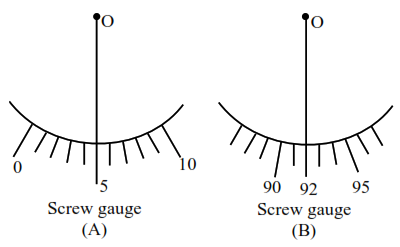# Student A and Student B

Question:

Student A and Student B used two screw gauges of equal pitch and 100 equal circular divisions to measure the radius of a given wire. The actual value of the radius of the wire is $0.322 \mathrm{~cm}$. The absolute value of the difference between the final circular scale readings observed by the students A and $B$ is

[Figure shows position of reference 'O' when jaws of screw gauge are closed]

Given pitch $=0.1 \mathrm{~cm}$.Solution:

For (A)

Reading $=$ MSR $+$ CSR $+$ Error

$0.322=0.300+\mathrm{CSR}+5 \times \mathrm{LC}$

$0.322=0.300+\mathrm{CSR}+0.005$

$\operatorname{CSR}=0.017$

For B

Reading $=$ MSR $+$ CSR $+$ Error

$0.322=0.200+\operatorname{CSR}+0.092$

$\mathrm{CSR}=0.030$

Difference $=0.030-0.017=0.013 \mathrm{~cm}$

Division on circular scale $=\frac{0.013}{0.001}=13$